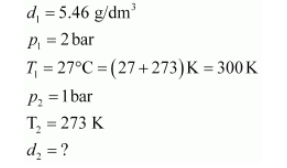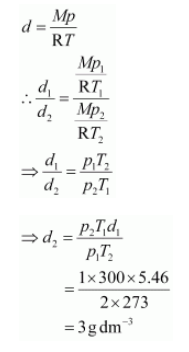# Density of a gas is found to be 5.46 g/dm3 at 27 °C at 2 bar pressure.`
Question:

Density of a gas is found to be $5.46 \mathrm{~g} / \mathrm{dm}^{3}$ at $27^{\circ} \mathrm{C}$ at 2 bar pressure. What will be its density at STP?

Solution:

Given,The density (d2) of the gas at STP can be calculated using the equation,Hence, the density of the gas at STP will be 3 g dm–3.